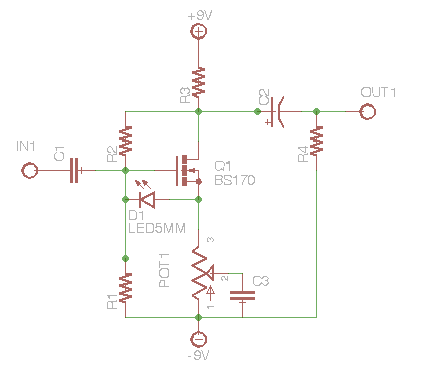# MosFET Range boost

Here’s a simple idea that you will work on almost every transistor
circuit out there. We’ll apply it to the MosFET boost here.

Remember the gain of the MosFET boost was determined by the Drain and
Source resistors. For the example circuit, as the Source resistor
decreases in value the gain increases.

A capacitor acts as a resistor when an AC signal is applied to it. The
resistance is dependent on the value of the cap and the frequency.
Lower frequencies see more resistance when the cap is smaller. This is
why you use a smaller cap to limit the amount of bass entering a
circuit. Or, use a larger cap to get more bass.

This idea can also be applied to the gain control to make a range
booster. Take a look at the attached file. Note that without C3 the
gain is 1 (assuming R3 and POT12  are equal). Add C3 to the wiper of
POT1 and you get a variable amount of gain to a frequency set by the
value of C3. Lower frequencies get attenuated.

When the wiper is at max resistance, you’re getting at least 5K before
the pot, so gain is 1. When the wiper is at minimum value the cap does
the variable resistance thing dependent on the frequency. Smaller cap
more highs get through. Higher frequencies see less resistance and
more gain, while lower frequencies see more resistance and less gain.

You can use this as an alternative to the SHO gain control by using a
large cap to boost a full range. Oops wait looks like Jack Orman did
it first. Take a look at his MosFET booster.Posted

in

by

Tags: